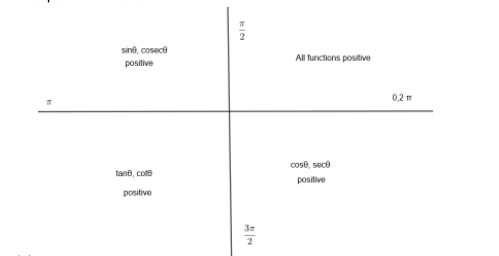Courses
Courses for Kids
Free study material
Free LIVE classes
MoreLIVE
Join Vedantu’s FREE Mastercalss

# If $\tan 2A=\cot \left( A-{{18}^{o}} \right)$where $2A$ is an acute angle, find the value of$A$.Verified
364.5k+ views
Hint: Relate $\tan \theta$and $\cot \theta$ by changing their angles
(complementary to each other).
Here, we have given condition for calculation of angle $A$ is
$\tan 2A=\cot \left( A-{{18}^{o}} \right).....\left( i \right)$
As we know that $\tan \theta$and $\cot \theta$, both are reciprocal functions and can be
related in the following way: $\tan \left( 90-\theta \right)=\cot \theta .....\left( ii \right)$
Now, we can use property $\left( ii \right)$with the equation $\left( i \right)$ to replace
$\cot \left( A-{{18}^{o}} \right)$
Let, $A-{{18}^{o}}=\theta .....\left( iii \right)$
So, the equation $\left( i \right)$becomes
$\tan 2A=\cot \theta$
Now, by using the equation $\left( i \right)$, we can put $\cot \theta =\tan \left( 90-\theta \right)$ in the following manner:
$\tan 2A=\cot \theta$
$\tan 2A=\tan \left( 90-\theta \right).....\left( iv \right)$
As we know that if $\tan {{\theta }_{1}}=\tan {{\theta }_{2}}$, then ${{\theta }_{1}}$ and
${{\theta }_{2}}$ must be equal to get the same tangent of angles.
Hence, from the equation $\left( iv \right)$, we can write
$2A=90-\theta$
Now, we already have $\theta =A-18$ from the equation $\left( iii \right)$.
Therefore, $2A=90-\left( A-18 \right)$
$2A=90-A+18$
$3A=108$
$A={{36}^{o}}$
As we already have given that $A$ is an acute angle, so $A={{36}^{o}}$ is correct.
Now, from $\tan {{\theta }_{1}}=\tan {{\theta }_{2}}$, we can get infinite solutions by writing
the general solution of it in the following way:
If $\tan {{\theta }_{1}}=\tan {{\theta }_{2}}$
${{\theta }_{1}}=n\pi +{{\theta }_{2}}$ where $n\in Z\left( n=0,1,2,3.... \right)$
Hence, we can calculate more numbers of angles $A$ but we need only an acute angle as
mentioned in the question. So, we need to find only the principle solution of $\tan {{\theta }_{1}}=\tan {{\theta }_{2}}$or
$\tan 2A=\tan \left( 108-A \right)$
$2A=n\pi +108-A$
$3A=n\pi +108$
$A=\dfrac{n\pi }{3}+36$; $n=0,1,2,3....$
For, $n=0,A={{36}^{o}}$ and for any other $n$, $A$ will be more than ${{90}^{o}}$. Hence
that will not be the correct solution for the given question.

Note: We can calculate angle $A$ by converting $\tan 2A$to $\cot$ function by using
$\tan \theta =\cot \left( 90-\theta \right)$
We have $\tan 2A=\cot \left( A-18 \right)$
$\cot \left( 90-2A \right)=\cot \left( A-18 \right)$
$90-2A=A-18$
$A={{36}^{o}}$
We can make a mistake when writing the equation $\tan \left( 90-\theta \right)=\cot \theta$. One can get confused in the conversion of one function to another. So, he/she must use

(1)(2) If the conversion is done like $\dfrac{n\pi }{2}+\theta$ type, then we need to change
$\tan \theta \rightleftarrows \cot \theta$
$\sin \theta \rightleftarrows \cos \theta$
$\text{cosec}\theta \rightleftarrows \sec \theta$
where $n$ is an odd integer.
If the conversion is like $\left( n\pi +\theta \right)$ type, then we need not convert any
function to others only taking care of signs should be involved.
Example:
(1) $\sin \left( \dfrac{\pi }{2}+\theta \right)=\cos \theta$
$\dfrac{\pi }{2}+\theta$will lie in the second quadrant, so $\sin$ will be positive.
(2) $\sin \left( \dfrac{3\pi }{2}+\theta \right)=-\cos \theta$
$\dfrac{3\pi }{2}+\theta$will lie in the ${{4}^{th}}$ quadrant.
(3) $\tan \left( 2\pi -\theta \right)=-\tan \theta$
$2\pi -\theta$will lie in the ${{4}^{th}}$ quadrant.

Last updated date: 29th Sep 2023
Total views: 364.5k
Views today: 8.64k